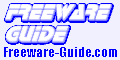PAST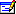PAST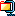3.9 MB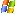all PAST is a free, easy-to-use data analysis package originally aimed at paleontology but now also popular in ecology and other fields. It includes common statistical, plotting and modelling functions: - A spreadsheet-type data entry form - Both interactive user interface and scripting - Graph, scatter, 3D scatter, bubble, histogram, kernel density estimation, box, percentile, ternary, survivorship, spindle, matrix, surface and normal probability plots - Curve fitting: Linear (Standard and Reduced Major Axis) with bootstrapping and permutation, lin-log (exponential), log-log (allometric), polynomial, logistic, von Bertalanffy, sum-of-sines, B-splines, Gaussian (species packing), multiple regression. - F, t, permutation t, Chi-squared w. permutation test, Fisher's exact, Kolmogorov-Smirnov, Mann-Whitney, Shapiro-Wilk, Jarque-Bera, Spearman's Rho and Kendall's Tau tests with permutation, correlation, covariance, contingency tables, one-way and two-way ANOVA, one-way ANCOVA, Kruskal-Wallis test, sign test, Wilcoxon signed rank test with permutation, Fligner-Killeen test for coefficients of variation, mixture analysis. - Diversity indices with bootstrapping and permutation, individual- and sample-based rarefaction. Capture-recapture richness estimators. Dice, Jaccard, Simpson and Raup-Crick similarity indices, Renyi diversity profiles, beta diversity. - Abundance model fitting: Geometric, log-series, log-normal, broken stick. - Multivariate statistics: Principal Components (with Minimal Spanning Tree, bootstrapping etc.), Principal Coordinates (19 distance measures), Non-metric Multidimensional Scaling (19 distance measures), Detrended Correspondence Analysis, Canonical Correspondence Analysis, Cluster analysis (UPGMA, single linkage, Ward's method and neighbour joining, 19 distance measures, two-way clustering, bootstrapping), k-means clustering, seriation, discriminant analysis, one-way MANOVA, one-way and two-way ANOSIM, one-way NPMANOVA, Hotelling's T2, paired Hotelling's T2, Mahalanobis-distance permutation, Mardia's multivariate normality, Box's M, Canonical Variates Analysis, multivariate allometry with bootstrapping, Mantel test, SIMPER, Imbrie & Kipp factor analysis, Modern Analog Technique, two-block Partial Least Squares. - Time series analysis: Spectral analysis, autocorrelation, cross-correlation, wavelet transform, Walsh transform, runs test. Mantel correlogram and periodogram. ARMA, Box-Jenkins intervention analysis. Solar forcing model. - Geometrical analysis: Directional statistics (Rayleigh, Rao, chi-squared, Watson-Williams, circular kernel density estimation, angular mean with CI, rose plots, circular correlation), point distribution statistics (nearest neighbour and Ripley's K), Fourier shape analysis, elliptic Fourier shape analysis, eigenshapes, landmark analysis with Bookstein and Procrustes fitting (2D and 3D), thin-plate spline transformation grids with expansions and principal strains, partial warps and scores, relative warps and scores, centroid size from landmarks, size removal by Burnaby's method. - Parsimony analysis (cladistics): Exhaustive, branch-and-bound and heuristic algorithms, Wagner, Fitch and Dollo characters. Bootstrap, strict and majority rule consensus trees. Consistency and retention indices. Three stratigraphic congruency indices with permutation tests. Cladograms and phylograms. - Biostratigraphy with the methods of Unitary Associations, Ranking-Scaling (RASC), Appearence Event Ordination and Constrained Optimization (CONOP). Confidence intervals on stratigraphic ranges. - Gridding (spatial interpolation): Moving average, thin-plate spline and kriging with three semivariogram models.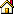Home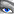Screenshot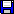Download

© The Freeware Guide